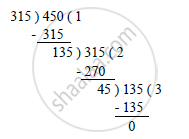# Find the Largest Number Which Divides 320 and 457 Leaving Remainders 5 and 7 Respectively. - Mathematics

Find the largest number which divides 320 and 457 leaving remainders 5 and 7 respectively.

#### Solution

We know that the required number divides 315 (320 – 5) and 450 (457 – 7).
∴ Required number = HCF (315, 450)
On applying Euclid’s lemma, we get:Therefore, the HCF of 315 and 450 is 45.
Hence, the required number is 45.

Concept: Euclid’s Division Lemma
Is there an error in this question or solution?